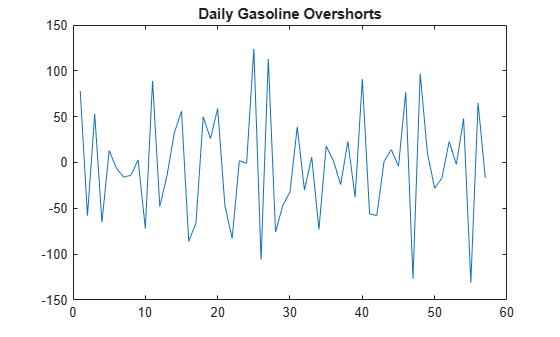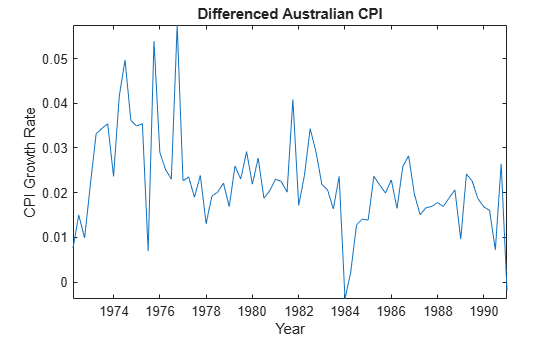# lbqtest

Ljung-Box Q-test for residual autocorrelation

## Syntax

``h = lbqtest(res)``
``h = lbqtest(res,Name,Value)``
``````[h,pValue] = lbqtest(___)``````
``````[h,pValue,stat,cValue] = lbqtest(___)``````

## Description

example

````h = lbqtest(res)` returns a logical value (`h`) with the rejection decision from conducting a Ljung-Box Q-Test for autocorrelation in the residual series `res`.```

example

````h = lbqtest(res,Name,Value)` uses additional options specified by one or more name-value pair arguments. If any name-value pair argument is a vector, then all name-value pair arguments specified must be vectors of equal length or length one. `lbqtest(res,Name,Value)` treats each element of a vector input as a separate test, and returns a vector of rejection decisions.If any name-value pair argument is a row vector, then `lbqtest(res,Name,Value)` returns a row vector. ```

example

``````[h,pValue] = lbqtest(___)``` returns the rejection decision and p-value for the hypothesis test, using any of the input arguments in the previous syntaxes.```

example

``````[h,pValue,stat,cValue] = lbqtest(___)``` additionally returns the test statistic (`stat`) and critical value (`cValue`) for the hypothesis test.```

## Examples

collapse all

Load the Deutschmark/British pound foreign-exchange rate data set.

`load Data_MarkPound`

Convert the prices to returns.

`returns = price2ret(Data);`

Compute the deviations of the return series.

`res = returns - mean(returns);`

Test the hypothesis that the residual series is not autocorrelated, using the default number of lags.

`h1 = lbqtest(res)`
```h1 = logical 0 ```

`h1 = 0` indicates that there is not enough evidence to reject the null hypothesis that the residuals of the returns are not autocorrelated.

Test the hypothesis that there are significant ARCH effects, using the default number of lags .

`h2 = lbqtest(res.^2)`
```h2 = logical 1 ```

`h2 = 1` indicates that there are significant ARCH effects in the residuals of the returns.

Test for residual heteroscedasticity using `archtest` and the default number of lags.

`h3 = archtest(res)`
```h3 = logical 1 ```

`h3 = 1` indicates that the null hypothesis of no residual heteroscedasticity should be rejected in favor of an ARCH(1) model. This result is consistent with `h2`.

Conduct multiple Ljung-Box Q-tests for autocorrelation by including various lags in the test statistic. The data set is a time series of 57 consecutive days of overshorts from an underground gasoline tank in Colorado . That is, the current overshort (${y}_{t}$) represents the accuracy in measuring the amount of fuel:

• In the tank at the end of day $t$

• In the tank at the end of day $t-1$

• Delivered to the tank on day $t$

• Sold on day $t$.

```load('Data_Overshort') y = Data; T = length(y); % Sample size figure plot(y) title('Overshorts for 57 Consecutive Days')````lbqtest` is appropriate for a series with a constant mean. Since the series appears to fluctuate around a constant mean, you do not need to transform the data.

Compute the residuals.

`res = y - mean(y);`

Assess whether the residuals are autocorrelated. Include 5, 10, and 15 lags in the test statistic computation.

`[h,pValue] = lbqtest(res,'lags',[5,10,15])`
```h = 1x3 logical array 1 1 1 ```
```pValue = 1×3 0.0016 0.0007 0.0013 ```

`h` and `pValue` are vectors containing three elements corresponding to tests at each of the three lags. The first element of each output corresponds to the test at lag 5, the second element corresponds to the test at lag 10, and the third element corresponds to the test at lag 15.

`h = 1` indicates the rejection of the null hypothesis that the residuals are not autocorrelated. `pValue` indicates the strength at which the test rejects the null hypothesis. Since all three $p-values$ are less than 0.01, there is strong evidence to reject the null hypothesis that the residuals are not autocorrelated.

Infer residuals from an estimated ARIMA model, and assess whether the residuals exhibit autocorrelation using `lbqtest`.

Load the Australian Consumer Price Index (CPI) data set. The time series (`cpi`) is the log quarterly CPI from 1972 to 1991. Remove the trend in the series by taking the first difference.

```load Data_JAustralian cpi = DataTable.PAU; T = length(cpi); dCPI = diff(cpi); figure plot(dates(2:T),dCPI) title('Differenced Australian CPI') xlabel('Year') ylabel('CPI growth rate') datetick axis tight```The differenced series appears stationary.

Fit an AR(1) model to the series, and then infer residuals from the estimated model.

```Mdl = arima(1,0,0); EstMdl = estimate(Mdl,dCPI);```
``` ARIMA(1,0,0) Model (Gaussian Distribution): Value StandardError TStatistic PValue _________ _____________ __________ __________ Constant 0.015564 0.0028766 5.4106 6.2808e-08 AR{1} 0.29646 0.11048 2.6834 0.0072876 Variance 0.0001038 1.1932e-05 8.6994 3.3362e-18 ```
```res = infer(EstMdl,dCPI); stdRes = res/sqrt(EstMdl.Variance); % Standardized residuals```

Assess whether the residuals are autocorrelated by conducting a Ljung-Box Q-test. The standardized residuals originate from the estimated model (`EstMdl`) containing parameters. When using such residuals, it is best practice to do the following:

• Adjust the degrees of freedom (`dof`) of the test statistic distribution to account for the estimated parameters.

• Set the number of lags to include in the test statistic.

• When you count the estimated parameters, skip the constant and variance parameters.

```lags = 10; dof = lags - 1; % One autoregressive parameter [h,pValue] = lbqtest(stdRes,'Lags',lags,'DOF',dof)```
```h = logical 1 ```
```pValue = 0.0119 ```

`pValue = 0.0130` suggests that there is significant autocorrelation in the residuals at the 5% level.

## Input Arguments

collapse all

Residual series for which the software computes the test statistic, specified as a numeric vector. The last element corresponds to the latest observation.

Typically, you fit a model to an observed time series, and `res` contains the standardized residuals from the fitted model.

Specify missing observations using `NaN`. The `lbqtest` function treats missing values as missing completely at random.

Data Types: `double`

### Name-Value Pair Arguments

Specify optional comma-separated pairs of `Name,Value` arguments. `Name` is the argument name and `Value` is the corresponding value. `Name` must appear inside quotes. You can specify several name and value pair arguments in any order as `Name1,Value1,...,NameN,ValueN`.

Example: `'Lags',1:4,'Alpha',0.1 ` specifies four tests with 1, 2, 3, and 4 lagged terms conducted at the 0.1 significance level.

Number of lagged terms to include in the test statistic calculation, specified as the comma-separated pair consisting of `'Lags'` and a positive integer or vector of positive integers.

Use a vector to conduct multiple tests.

Each element of `Lags` must be less than `T`, which is the effective sample size of `res` (that is, the number of nonmissing values in `res`).

Example: `'Lags',1:4`

Data Types: `double`

Significance levels for the hypothesis tests, specified as the comma-separated pair consisting of `'Alpha'` and a scalar or vector.

Use a vector to conduct multiple tests.

Each element of `Alpha` must be greater than 0 and less than 1.

Example: `'Alpha',0.01`

Data Types: `double`

Degrees of freedom for the asymptotic, chi-square distribution of the test statistics, specified as the comma-separated pair consisting of `'DoF'` and a positive integer or vector of positive integers.

Use a vector to conduct multiple tests.

If `DoF` is an integer, then it must be less than or equal to `Lags`. Otherwise, each element of `DoF` must be less than or equal to the corresponding element of `Lags`.

Example: `'DoF',15`

Data Types: `double`

## Output Arguments

collapse all

Test rejection decisions, returned as a logical value or vector of logical values with a length equal to the number of tests that the software conducts.

• `h = 1` indicates rejection of the no residual autocorrelation null hypothesis in favor of the alternative.

• `h = 0` indicates failure to reject the no residual autocorrelation null hypothesis.

Test statistic p-values, returned as a scalar or vector with a length equal to the number of tests that the software conducts.

Test statistics, returned as a scalar or vector with a length equal to the number of tests that the software conducts.

Critical values determined by `Alpha`, returned as a scalar or vector with a length equal to the number of tests that the software conducts.

collapse all

### Ljung-Box Q-Test

The Ljung-Box Q-test is a “portmanteau” test that assesses the null hypothesis that a series of residuals exhibits no autocorrelation for a fixed number of lags L, against the alternative that some autocorrelation coefficient ρ(k), k = 1, ..., L, is nonzero.

The test statistic is

`$Q=T\left(T+2\right)\sum _{k=1}^{L}\left(\frac{\rho {\left(k\right)}^{2}}{\left(T-k\right)}\right),$`

where T is the sample size, L is the number of autocorrelation lags, and ρ(k) is the sample autocorrelation at lag k. Under the null hypothesis, the asymptotic distribution of Q is chi-square with L degrees of freedom.

### Missing Completely at Random

Observations of a random variable are missing completely at random if the tendency of an observation to be missing is independent of both the random variable and the tendency of all other observations to be missing.

## Tips

If you obtain `res` by fitting a model to data, then you should reduce the degrees of freedom (the argument `DoF`) by the number of estimated coefficients, excluding constants. For example, if you obtain `res` by fitting an `ARMA`(p,q) model, set `DoF` to Lpq, where L is `Lags`.

## Algorithms

• The `Lags` argument affects the power of the test.

• If L is too small, then the test does not detect high-order autocorrelations.

• If L is too large, then the test loses power when a significant correlation at one lag is washed out by insignificant correlations at other lags.

• Box, Jenkins, and Reinsel suggest setting `min[20,T-1]` as the default value for `lags` .

• Tsay cites simulation evidence that setting `lags` to a value approximating log(T) provides better power performance .

• `lbqtest` does not directly test for serial dependencies other than autocorrelation. However, you can use it to identify conditional heteroscedasticity (ARCH effects) by testing squared residuals .

Engle's test assesses the significance of ARCH effects directly. For details, see `archtest`.

 Box, G. E. P., G. M. Jenkins, and G. C. Reinsel. Time Series Analysis: Forecasting and Control. 3rd ed. Englewood Cliffs, NJ: Prentice Hall, 1994.

 Brockwell, P. J. and R. A. Davis. Introduction to Time Series and Forecasting. 2nd ed. New York, NY: Springer, 2002.

 Gourieroux, C. ARCH Models and Financial Applications. New York: Springer-Verlag, 1997.

 McLeod, A. I. and W. K. Li. "Diagnostic Checking ARMA Time Series Models Using Squared-Residual Autocorrelations." Journal of Time Series Analysis. Vol. 4, 1983, pp. 269–273.

 Tsay, R. S. Analysis of Financial Time Series. 2nd Ed. Hoboken, NJ: John Wiley & Sons, Inc., 2005.